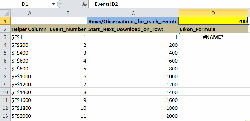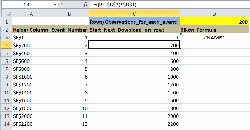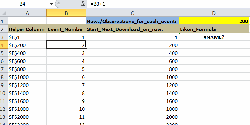## Thomson Eikon & Event windowsNot too long ago I was working with the successor of Thomson 1 / Thomson One which is called Eikon or Thomson Eikon. This product is web-based and is similar in look and style as Bloomberg. I found out that in most cases for me the Excel add-in was the most useful part and allowed me to download larger amounts of data.
The specific Add-in did, however, miss a key feature which I use heavily in Datastream: there is no Request Table tool to allow event studies with changing dates and time windows. Using the add-in I was able to figure out how to still do something like this using the commands which Thomson inputs in your Excel worksheet to call up the data.

With a bit of help from the Eikon Helpdesk I was able to come up with a template that kind of does the same thing as a Request Table Excel tool from Datastream. You can download the example here.

Explanation of the worksheet:

0) Before using the worksheet template, make sure that the connection to Eikon on your computer is not yet live. Otherwise the sheet will immediately start updating as the Add-in and Eikon code is usually set up this way.

1) The first sheet is called Events
As the name already suggests, this is where you put the codes for the series (column A). In columns B and C the Start and End dates for the series go. If you need an exact number of trading days (of data) you can use the formulas from a previous post.
If the exact number of days need not be the exact same column D shows the number of calendar days between the start and end days. This is done by deducting the end date from the start date. This works fine as dates are also numbers in Excel. Cell E2 shows the highest number in the entire column D using formula =MAX(D:D)2) The second sheet is Data
The example sheet is used to download the Eikon data into and is based on generated Eikon code(s).

Cell D1 in sheet Data is linked to Cell E2 from the Events sheet and will show the same value with the formula: =Events!D2
This number is important as this indicates the (maximum) number of rows for each new window to start downloading data without overwriting the data from previous (downloaded) series. This may cause empty rows between series/data, but these empty rows can be deleted afterwardsThe formulas in Column C are used to calculate the row/cell numbers for each separate series to start downloading: =((B4-\$B\$3)*\$D\$1)This is also based on the generated number of events in columb B: =B3+1.The Helper Column is column A. This column is needed to get the download destinations to be included later in the Cells with the Thomson Eikon codes. There is just a technical reason for this, otherwise the Eikon code does not accept the generated destination cells. The formula is: =ADDRESS(\$C4;6) where the \$C4 is the number of rows it takes from column C and 6 indicates the column number where the download should start.The download code from eikon is as follows: =TR(Events!\$A2;”TR.PriceClose”;”Frq=D SDate=#1 EDate=#2 Curn=EUR CH=Fd;date;IN Transpose=Y NULL=NA CODE=ISIN”;INDIRECT(\$A3);Events!\$B2;Events!\$C2)• This formula takes the series data from the Events tab in column A where it says Events!\$A2
• The start & end dates are included at the end where it says: Events!\$B2;Events!\$C2
• The data download cell destinations for the events are indicated where it says: INDIRECT(\$A3)

You just copy everything downwards (including the Eikon formula) depending on the number of events in the dataset.

3) When you are done copying the events in to the sheet Events and copying the necessary formulas in the Data sheet, you can then go live by signing in into Eikon. You will see that the data download starts automatically.

N.B.: The example download sheet downloads the closing price for series with the Eikon datatype code TR.PriceClose. If you need another datatype this part needs to be changed in the formula.Email